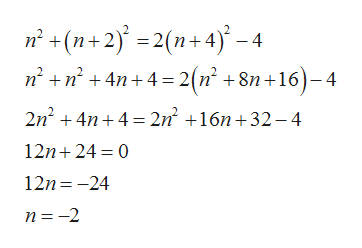Find three consecutive even integers such that the sum of the squares of the first two is four less than twice the square of the third.

Question

Find three consecutive even integers such that the sum of the squares of the first two is four less than twice the square of the third.

Step 1

Let the three consecutive even integers are n , n+2, n+4

Step 2

The sum of square of n and sqaure of n+2 is four less than twice the square of n+4help_outlineImage Transcriptionclosen (n+2)2(n+4)-4 nn4n4 = 2(n' +8n+16-4 2n24n4 2n2 16n+32-4 12n 24 0 12n 24 n -2 fullscreen
Step 3

Thus the numbers are n=-2. n+2=-2...

Want to see the full answer?

See Solution

Want to see this answer and more?

Our solutions are written by experts, many with advanced degrees, and available 24/7

See Solution
Tagged in

Equations and In-equations